# Price Elasticity of Demand (PED)

Updated February 2, 2021

## What Is PED?

Price elasticity of demand (PED) measures the change in the demand for a product or service in response to a change in its price.

With most goods, an increase in price leads to a decrease in demand – and a decrease in price leads to an increase in demand. When there is a large change in demand after a price change, that good is considered to have "elastic demand." On the other hand, if there is only a small change in demand, that good is considered to have relatively inelastic demand.

Price elasticity of demand measures the responsiveness of demand, highlighting whether a good is elastic or inelastic.

## How to Calculate Price Elasticity of Demand

PED is calculated by dividing the percentage change in quantity demanded by the percentage change in price. The value resulting from that calculation indicates the responsiveness of demand.

### PED Formula

The formula for price elasticity of demand is: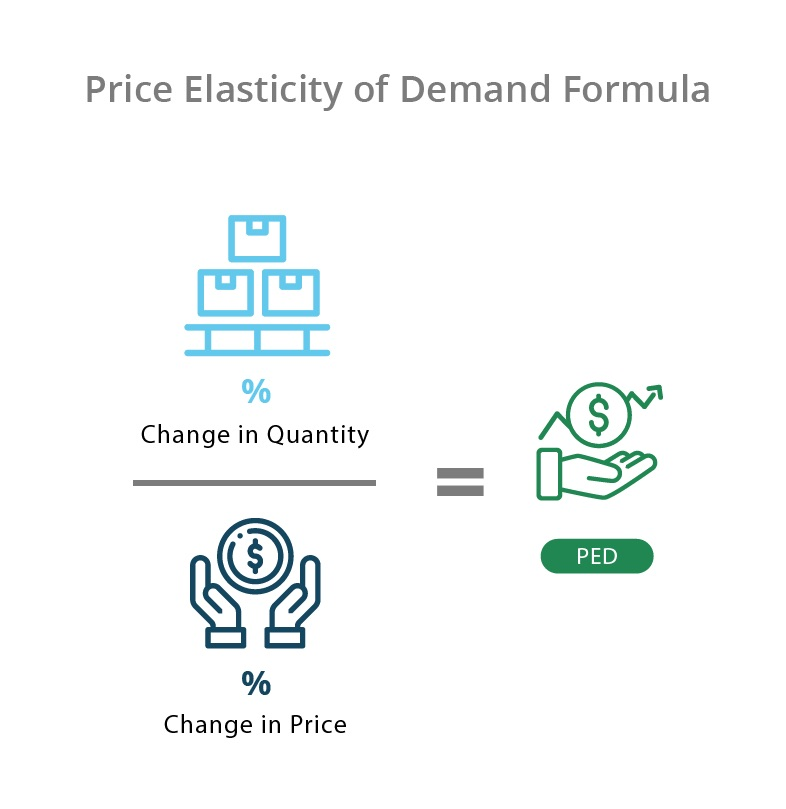If you don't have the percentage changes in quantity and price, use the following formula instead: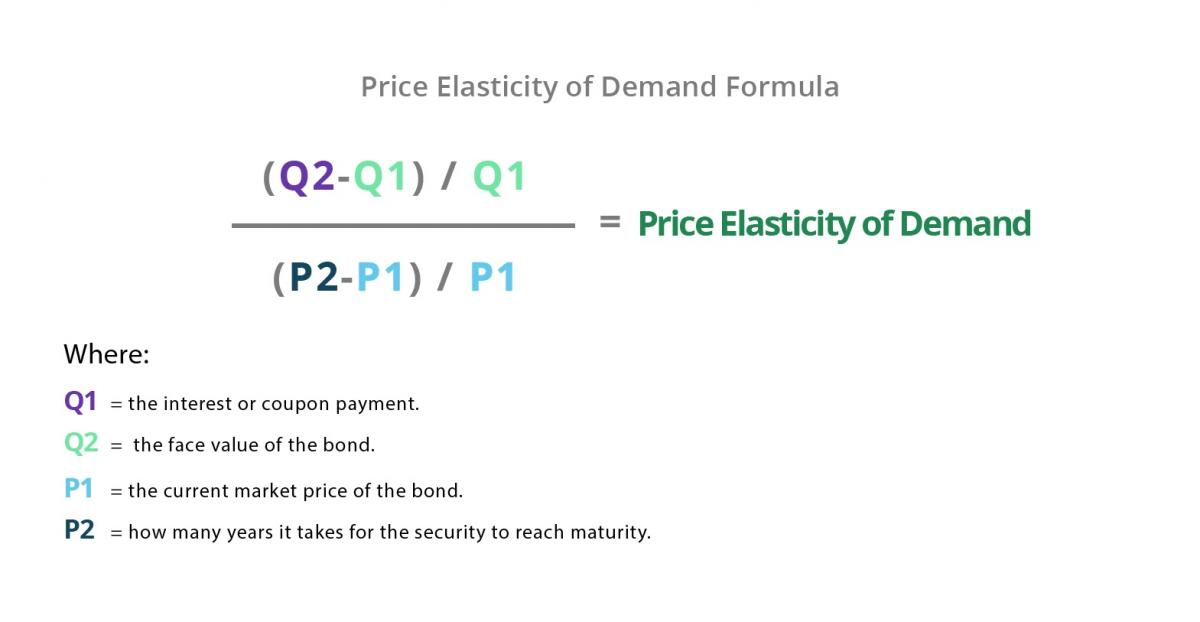## Is Price Elasticity of Demand Elastic or Inelastic?

You can tell if price elasticity of demand is elastic or inelastic from the value provided by the price elasticity of demand formula. If the value from that equation is: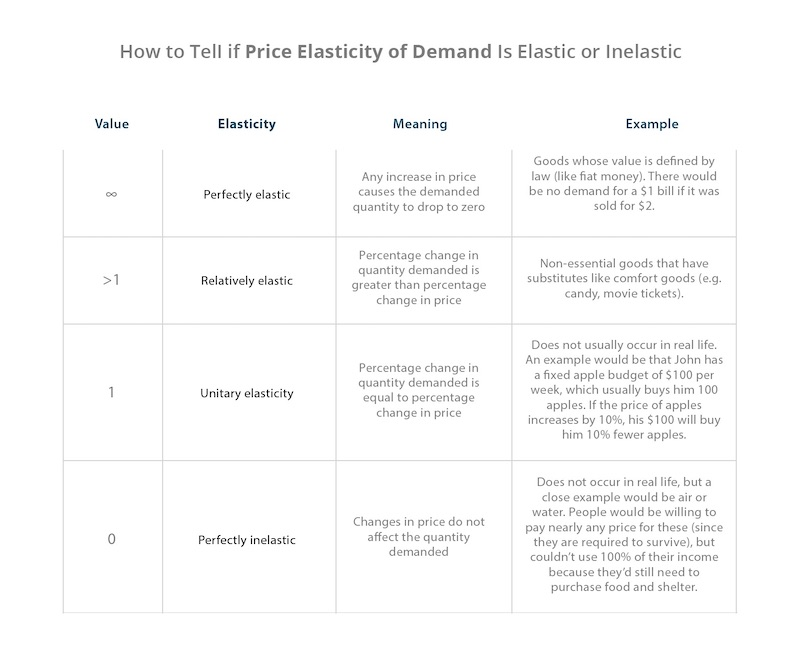## Price Elasticity of Demand Examples

The PED calculations above will give you a number that indicates whether demand for a good is elastic or inelastic:

• If the demand for a good is elastic, the change in demand is greater than the change in price.
• If it’s inelastic, the change in demand is smaller than the change in price.

### Elastic Price Elasticity of Demand Example

There are 100 people who need to get home on New Year’s Eve and the average cost per ride is \$20. At this rate, all people are willing to pay that cost to get home.

Uber’s surge pricing raises the average cost to \$30 per ride (a price increase of 50%). However, most people don’t live too far from home, so 75 of them decide to walk. This leaves only 25 people who call ubers (a 75% decrease in demand). To calculate price elasticity of demand, you use the formula from above: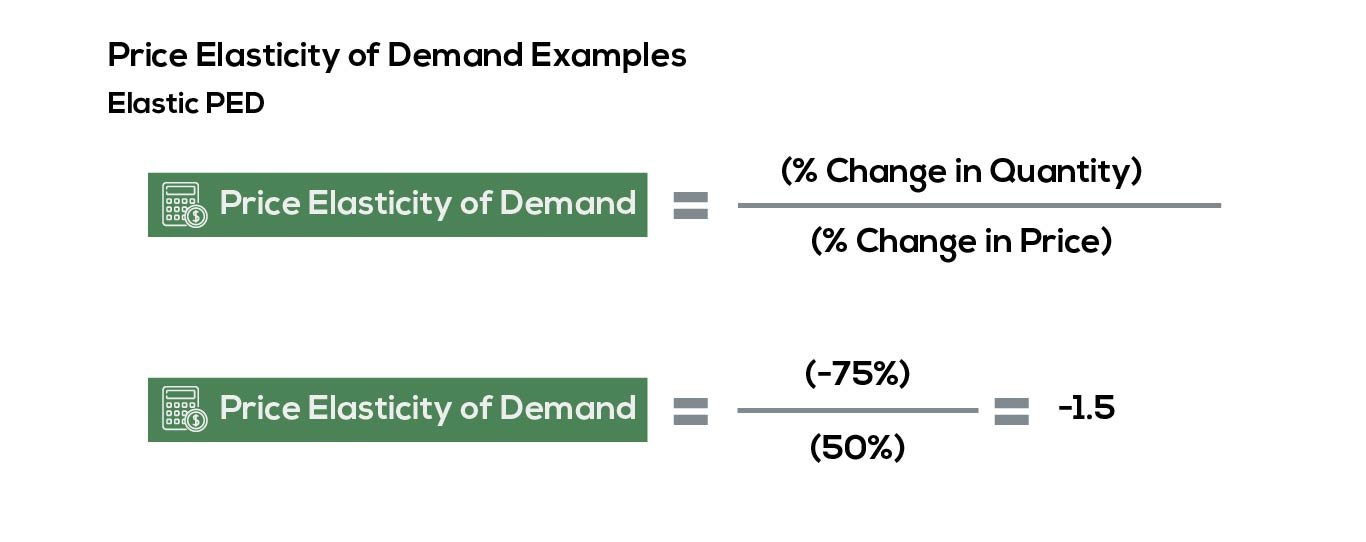Since the equation uses absolute value (omits the negative sign), the price elasticity of demand in this situation would be 1.5. This means that for every 1% increase in price, there is a 1.5% decrease in demand. Since the change in demand is greater than the change in price, we can conclude that demand is relatively elastic.

### Inelastic Price Elasticity of Demand Example

Once again, there are 100 people who need to get home on New Year’s Eve. The average cost per ride is \$20. At this rate, all people are willing to pay this cost.

Assume that Uber’s surge pricing kicks in, raising the average cost to \$30 per ride (a price increase of 50%). 25 of them end up calling friends or family instead, leaving only 75 people to call Ubers (a decrease in demand of 25%). To calculate price elasticity of demand, you use the formula from above: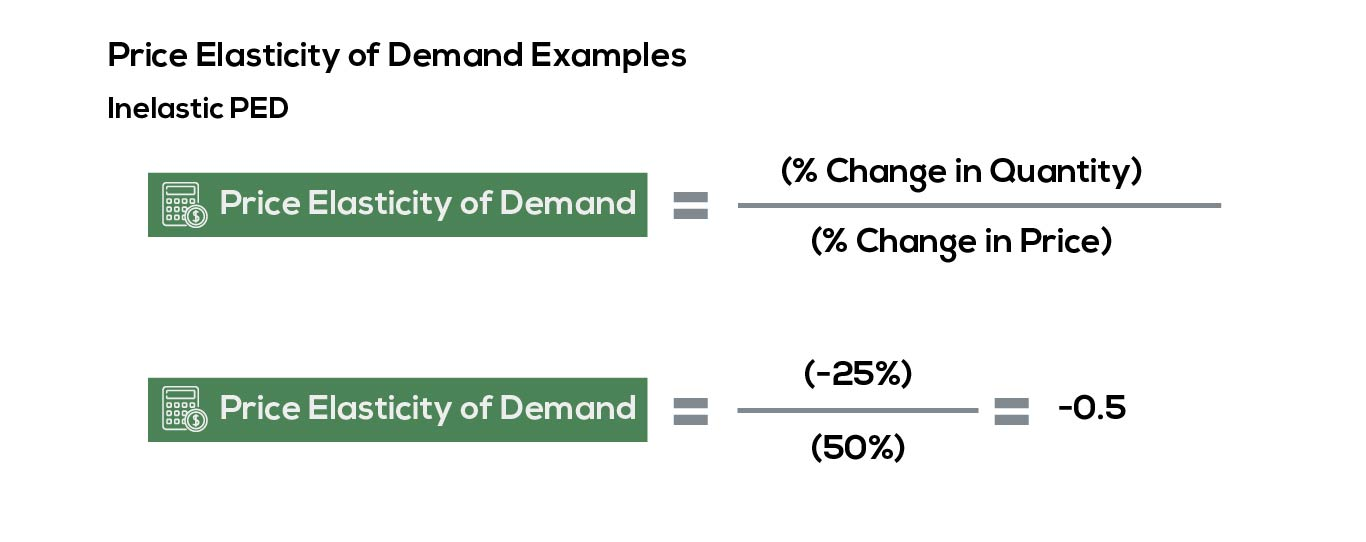The price elasticity of demand in this situation would be 0.5 or 0.5%. This means that for every 1% increase in price, there is a 0.5% decrease in demand. Since the change in demand is smaller than the change in price, we can conclude that demand is relatively inelastic.

## Negative Elasticity: What Does It Mean?

Generally speaking, demand will decrease when price increases, and demand will increase when price decreases. That means that the price elasticity of demand is almost always negative (since demand and price have an inverse relationship). Because there is almost always one decreasing variable, the resulting value will be negative.

It’s important to note that price elasticity of demand uses absolute value (meaning that it essentially ignores the negative symbol). Since we already know the direction demand will shift, we’re more concerned about the value of the number since it explains whether demand is elastic or inelastic  – and how responsive it is to changes in price.

## What Is The Difference Between Price Elasticity of Demand and Price Elasticity of Supply?

Price elasticity of demand measures the responsiveness of demand resulting from a change in price. Price elasticity of supply, however, measures the change in the supply of a good when there is a change in its price.

All of our content is verified for accuracy by Rachel Siegel, CFA and our team of certified financial experts. We pride ourselves on quality, research, and transparency, and we value your feedback. Below you'll find answers to some of the most common reader questions about Price Elasticity of Demand (PED).
##### Be the first to ask a question

Rachel Siegel, CFA - 141Rachel Siegel, CFACFA Charterholder

Chartered Financial Analyst

Rachel Siegel, CFA is one of the nation's leading experts at ensuring the accuracy of financial and economic text.  Her prestigious background includes over 10 years creating professional financial certification exams and another 20 years of college-level teaching.